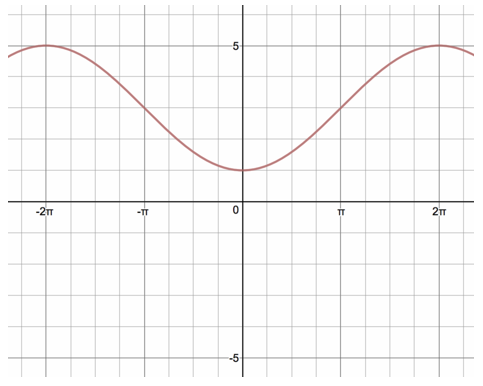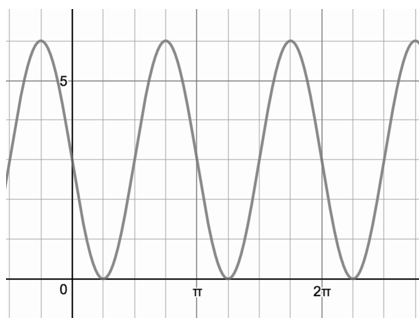# Unit Circle and Trigonometric Functions

## Objective

Graph transformations of sine and cosine functions (Part II).

## Common Core Standards

### Core Standards

?

• F.BF.B.3 — Identify the effect on the graph of replacing f(x) by f(x) + k, k f(x), f(kx), and f(x + k) for specific values of k (both positive and negative); find the value of k given the graphs. Experiment with cases and illustrate an explanation of the effects on the graph using technology. Include recognizing even and odd functions from their graphs and algebraic expressions for them.

• F.IF.C.7.E — Graph exponential and logarithmic functions, showing intercepts and end behavior, and trigonometric functions, showing period, midline, and amplitude.

## Criteria for Success

?

1. Identify period, amplitude, midline, and phase shift of sine and cosine functions.
2. Graph sine and cosine functions from equations.
3. Write equations for graphs of sine and cosine functions.
4. Write equations in multiple forms using phase shifts and both sine and cosine functions.

## Tips for Teachers

?

• Students will need computers for this lesson. The Desmos activity will be best done by individual students rather than as a class demonstration.
• This lesson will require lots of practice, but students will see and practice these topics again in future lessons.

## Anchor Problems

?

### Problem 1

Show students Graph 21 from Which One Doesn't Belong: Graphs and Equations.

#### References

Which One Doesn't Belong Which One Doesn't BelongGraph 21

### Problem 2

Do this Desmos activity, Sine Transformations, with students.

#### References

Desmos Sine Transformations

Sine Transformations by Jeremy Babel is made available by Desmos. Copyright © 2017 Desmos, Inc. Accessed Feb. 26, 2018, 3:43 p.m..

### Problem 3

Write both a sine and cosine function that model the graph shown below.## Problem Set

?

The following resources include problems and activities aligned to the objective of the lesson that can be used to create your own problem set.

• "If you were given the following two points and told that they were the maximum and minimum of sine function, how would you write the equation of the sinusoidal graph?"
• Include problems where students look at graphs of similar sinusoidal functions and have them identify what has changed in each.
• Ensure that students are using the vocabulary of:
• domain
• range
• period
• amplitude
• midline
• maximum
• minimum
• Include problems giving an equation, asking to describe the features of the sinusoidal function.
• Include problems sketching graphs, focused on two or three transformations for each graph.
• Include problems giving a graph and asking to write an equation.
• "What horizontal shifts would result in a cosine function?"
• "If given a cosine function, what horizontal shifts would result in a sine function?"
• Include problems identifying missing values on equations.
• Error analysis where graph is mismatched to the equation of the sine function.
• Desmos Polygraph: SinusoidsUse as is with students or pull apart the graphs for a paper modification${y=-3\mathrm{sin}(2x)+3}$